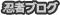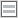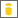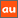# 0除算に関するアナウンスメントを公開しています

The purpose of this site is to publish announcements on the theory of division by zero

## Announcement 300: New challenges on the division by zero $z/0=0$

Institute of Reproducing Kernels
Kawauchi-cho, 5-1648-16,
Kiryu 376-0041, Japan
E-mail: kbdmm360@yahoo.co.jp
May 22, 2016

Abstract: In this announcement, for its importance we would like to state the
situation on the division by zero and propose basic new challenges.

1 Introduction
By a natural extension of the fractions
\begin{equation}
\frac{b}{a} \tag{1.1}
\end{equation}for any complex numbers $a$ and $b$, we found the result, for any complex number $b$
\begin{equation}
\frac{b}{0}=0, \tag{1.2}
\end{equation}incidentally in  by the Tikhonov regularization for the Hadamard product inversions for matrices, and we discussed their properties and gave several physical interpretations on the general fractions in  for the case of real numbers.
The division by zero has a long and mysterious story over the world $($see, for example, Google site with division by zero$)$ with its physical viewpoints since the document of zero in India on AD 628, however, Sin-Ei, Takahasi   established a simple and decisive interpretation $($1.2$)$ by analyzing some full extensions of fractions and by showing the complete characterization for the property $($1.2$)$:

Proposition. Let F be a function from ${\bf C }\times {\bf C }$ to ${\bf C }$ satisfying
\begin{equation}
F (b, a)F (c, d)= F (bc, ad)
\end{equation}for all $a, b, c, d \in {\bf C }$, and
$$F (b, a) = \frac {b}{a }, \quad a, b \in {\bf C }, a \ne 0.$$Then, we obtain, for any $b \in {\bf C }$
$F (b, 0) = 0.$
Note that the complete proof of this proposition is simply given by  2 or 3 lines.
We thus should consider, for any complex number $b$, as  $($1.2$)$; that is, for the mapping
\begin{equation}
w = \frac{1}{z}, \tag{1.3}
\end{equation}the image of $z=0$ is $w=0$ $($should be defined$)$. This fact seems to be a curious one in connection with our well-established popular image for the  point at infinity on the Riemann sphere. Therefore, the division by zero will give great impacts to complex analysis and to our ideas for the space and universe.
However, the division by zero $($1.2$)$ is now clear, indeed, for the introduction of $($1.2$)$, we have several independent approaches as in:
1) by the generalization of the fractions by the Tikhonov regularization or by the Moore-Penrose generalized inverse,
2) by the intuitive meaning of the fractions $($division$)$ by H. Michiwaki,
3) by the unique extension of the fractions by S. Takahasi,   as in the above,
4) by the extension of the fundamental function $W = 1/z$ from ${\bf C} \setminus \{0\}$ into ${\bf C}$ such that $W =1/z$ is a one to one and onto mapping from ${\bf C} \setminus \{0\}$ onto ${\bf C} \setminus \{0\}$ and the division by zero $1/0=0$ is a one to one and onto mapping extension of the function $W =1/z$ from  ${\bf C}$ onto ${\bf C}$,
and
5) by considering the values of functions with the mean values of functions.
Furthermore, in  we gave the results in order to show the reality of the division by zero in our world:
A) a field structure  containing the division by zero --- the Yamada field ${\bf Y}$,
B)  by the gradient of the $y$ axis on the $(x,y)$ plane --- $\tan \frac{\pi}{2} =0$,
C) by the reflection $W =1/\overline{z}$ of $W= z$ with respect to the unit circle with center at the origin on the complex $z$ plane --- the reflection point of zero is zero,
and
D) by considering rotation of a right circular cone having some very interesting phenomenon  from some practical and physical problem.
In ,  many division by zero results in Euclidean spaces are given and  the basic idea at the point at infinity should be changed. In , we gave beautiful geometrical interpretations of determinants from the viewpoint of the division by zero. The results show that the division by zero is our basic and elementary mathematics in our world.
See  J. A. Bergstra, Y. Hirshfeld and J. V. Tucker  for the relationship between fields and the division by zero, and the importance of the division by zero for computer science. It seems that the relationship of the division by zero and field structures are abstract in their paper.
Meanwhile,  J. P.  Barukcic and I.  Barukcic  discussed recently the relation between the divisions $0/0$, $1/0$ and special relative theory of Einstein. However, their logic seems to be curious and their results contradict with ours.
Furthermore,  T. S. Reis and J.A.D.W. Anderson [8,9] extend the system of the real numbers by introducing an ideal number for the division by zero $0/0$.
Meanwhile, we should refer to up-to-date information:
Kurt Arbenz
https://www.researchgate.net/publication/272022137 Riemann Hypothesis Addendum -   Breakthrough.
Here, we recall Albert Einstein's words on mathematics: Blackholes are where God divided by zero. I don't believe in mathematics. George Gamow $($1904-1968$)$ Russian-born American nuclear physicist and cosmologist remarked that "it is well known to students of high school algebra" that division by zero is not valid; and Einstein admitted it as the biggest blunder of his life  1. Gamow, G., My World Line $($Viking, New York$)$. p 44, 1970.
For our ideas on the division by zero, see the survey style announcements 179, 185, 237, 246,247, 250 and 252 of the Institute of Reproducing Kernels [12, 13, 14, 15, 16, 17, 18, 21].

2 On mathematics
Apparently, the division by zero is a great missing in our mathematics and the result $($1.2$)$ is definitely determined as our basic mathematics, as we see from Proposition 1.  Note  its very general assumptions and  many fundamental evidences in our world in [4, 6, 7]. The results will give great impacts  on Euclidean spaces, analytic geometry, calculus, differential equations, complex analysis and  physical problems. See our announcements for the details.
The mysterious history of the division by zero over one thousand years is a great shame of  mathematicians and human race on the world history, like the Ptolemaic system $($geocentric theory$)$. The division by zero will become a typical  symbol of foolish human race with long and unceasing struggles. Future people will realize this fact as a definite common sense.
We should check and fill our mathematics, globally and beautifully, from the viewpoint of the division by zero. Our mathematics will be more perfect and beautiful,  and will give great impacts to our basic ideas on the universe.

3. Albert Einstein's biggest blunder
The division by zero is directly related to the Einstein's theory and various physical problems
containing the division by zero.  Now we should check the theory and the problems by the concept of the RIGHT and DEFINITE division by zero. Now is the best time since 100 years from Albert Einstein. It seems that the background knowledge is timely fruitful.

4. Computer systems
The above Professors listed are wishing the contributions in order to avoid the zero division trouble in computers. Now,  we should arrange  new computer systems in order not to meet the division by zero trouble in computer systems.

5. General  ideas on the universe
The division by zero may be related to religion,  philosophy and the ideas on the universe, and it will creat a new world. Look the new world.
We are standing on a new  generation and in front of the new world, as in the discovery of the Americas.

References
 J. P.  Barukcic and I.  Barukcic, Anti Aristotle - The Division of Zero by Zero. Journal of Applied Mathematics and Physics,   4 $($2016$)$, 749-761. doi: 10.4236/jamp.2016.44085.
 J. A. Bergstra, Y. Hirshfeld and J. V. Tucker, Meadows and the equational specification of division $($arXiv:0901.0823v1[math.RA] 7 Jan 2009$)$.
 L. P.  Castro and S. Saitoh,  Fractional functions and their representations,  Complex Anal. Oper. Theory 7 $($2013$)$, no. 4, 1049-1063.
 M. Kuroda, H. Michiwaki, S. Saitoh, and M. Yamane, New meanings of the division by zero and interpretations on $100/0=0$ and on $0/0=0$,
Int. J. Appl. Math.  27 $($2014$)$, no 2, pp. 191-198,  DOI: 10.12732/ijam.v27i2.9.
 T. Matsuura and S. Saitoh, Matrices and division by zero $z/0=0$, Linear Algebra \& Matrix Theory $($ALAMT$)$ $($to appear$)$.
 H. Michiwaki, S. Saitoh,  and  M.Yamada, Reality of the division by zero $z/0=0$.  IJAPM  International J. of Applied Physics and Math.  6 $($2015$)$, 1--8. http://www.ijapm.org/show-63-504-1.html
 H.  Michiwaki, H. Okumura, and S. Saitoh, Division by Zero $z/0 = 0$ in Euclidean Spaces. International Journal of Mathematics and Computation  $($in press$)$.
 T. S. Reis and J.A.D.W. Anderson, Transdifferential and Transintegral Calculus, Proceedings of the World Congress on Engineering and Computer Science 2014 Vol I WCECS 2014, 22-24 October, 2014, San Francisco, USA
 T. S. Reis and J.A.D.W. Anderson, Transreal Calculus, IAENG  International J. of Applied Math.,  45 $($2015$)$:  IJAM 45 1 06.
 S. Saitoh, Generalized inversions of Hadamard and tensor products for matrices,  Advances in Linear Algebra \& Matrix Theory.  4  $($2014$)$, no. 2,  87--95. http://www.scirp.org/journal/ALAMT/
 S.-E. Takahasi, M. Tsukada and Y. Kobayashi,  Classification of continuous fractional binary operations on the real and complex fields,  Tokyo Journal of Mathematics,   38 $($2015$)$, no. 2, 369-380.
 Announcement 179 $($2014.8.30$)$: Division by zero is clear as $z/0=0$ and it is fundamental in mathematics.
 Announcement 185 $($2014.10.22$)$: The importance of the division by zero $z/0=0$.
 Announcement 237 $($2015.6.18$)$:  A reality of the division by zero $z/0=0$ by  geometrical optics.
 Announcement 246 $($2015.9.17$)$: An interpretation of the division by zero $1/0=0$ by the gradients of lines.
 Announcement 247 $($2015.9.22$)$: The gradient of y-axis is zero and $\tan (\pi/2) =0$ by the division by zero $1/0=0$.
 Announcement 250 $($2015.10.20$)$: What are numbers? -  the Yamada field containing the division by zero $z/0=0$.
 Announcement 252 $($2015.11.1$)$: Circles and curvature - an interpretation by Mr. Hiroshi Michiwaki of the division by zero $r/0 = 0$.
 Announcement 281$($2016.2.1$)$: The importance of the division by zero $z/0=0$.
 Announcement 282$($2016.2.2$)$: The Division by Zero $z/0=0$ on the Second Birthday.
 Announcement 293$($2016.3.27$)$:  Parallel lines on the Euclidean plane from the viewpoint of division by zero 1/0=0.

PR

### コメント

お名前 Black LimeGreen SeaGreen Teal FireBrick Tomato IndianRed BurlyWood SlateGray DarkSlateBlue LightPink DeepPink DarkOrange Gold DimGray Silverプロフィール

ＨＮ：

(01/17)
(01/14)
(01/12)
(01/04)
(01/03)